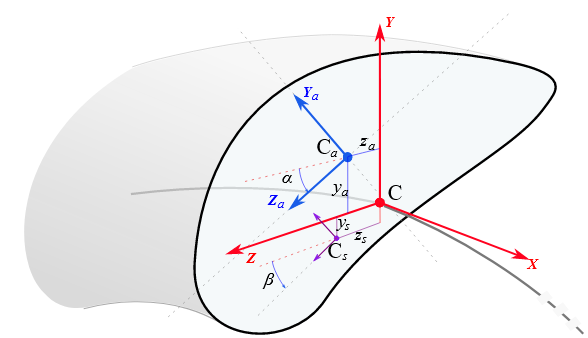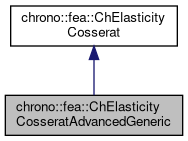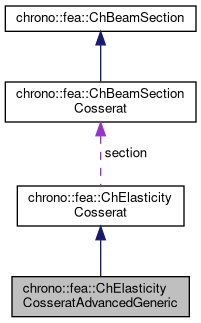## Description

Advanced linear elasticity for a Cosserat section, not assuming homogeneous elasticity.

This is the case where one uses a FEA preprocessor to compute the rigidity of a complex beam made with multi-layered reinforcements with different elasticity - in such a case you could not use ChElasticityCosseratAdvanced because you do not have a single E or G, but you rather have collective values of bending/shear/axial rigidities. This class allows using these values directly, bypassing any knowledge of area, Izz Iyy, E young modulus, etc. This material can be shared between multiple beams. The linear elasticity is uncoupled between shear terms S and axial terms A as to have this stiffness matrix pattern:

 n_x   [A       A A ]   e_x
n_y   [  S S S     ]   e_y
n_z = [  S S S     ] * e_z
m_x   [  S S S     ]   k_x
m_y   [A       A A ]   k_y
m_z   [A       A A ]   k_z#include <ChBeamSectionCosserat.h>[legend][legend]

## Public Member Functions

ChElasticityCosseratAdvancedGeneric (const double mAx, const double mTxx, const double mByy, const double mBzz, const double mHyy, const double mHzz, const double malpha, const double mCy, const double mCz, const double mbeta, const double mSy, const double mSz)

virtual void SetAxialRigidity (const double mv)
Sets the axial rigidity, usually A*E for uniform elasticity, but for nonuniform elasticity here you can put a value ad-hoc from a preprocessor.

virtual void SetXtorsionRigidity (const double mv)
Sets the torsion rigidity, for torsion about X axis, at elastic center, usually J*G for uniform elasticity, but for nonuniform elasticity here you can put a value ad-hoc from a preprocessor

virtual void SetYbendingRigidity (const double mv)
Sets the bending rigidity, for bending about Y axis, at elastic center, usually Iyy*E for uniform elasticity, but for nonuniform elasticity here you can put a value ad-hoc from a preprocessor.

virtual void SetZbendingRigidity (const double mv)
Sets the bending rigidity, for bending about Z axis, at elastic center, usually Izz*E for uniform elasticity, but for nonuniform elasticity here you can put a value ad-hoc from a preprocessor.

virtual void SetYshearRigidity (const double mv)
Sets the shear rigidity, for shear about Y axis, at shear center, usually A*G*(Timoshenko correction factor) for uniform elasticity, but for nonuniform elasticity here you can put a value ad-hoc from a preprocessor.

virtual void SetZshearRigidity (const double mv)
Sets the shear rigidity, for shear about Z axis, at shear center, usually A*G*(Timoshenko correction factor) for uniform elasticity, but for nonuniform elasticity here you can put a value ad-hoc from a preprocessor.

void SetSectionRotation (double ma)
Set the rotation in [rad] of the Y Z axes for which the YbendingRigidity and ZbendingRigidity values are defined. More...

double GetSectionRotation ()

void SetCentroid (double my, double mz)
"Elastic reference": set the displacement of the elastic center (or tension center) respect to the reference section coordinate system placed at centerline.

double GetCentroidY ()

double GetCentroidZ ()

void SetShearRotation (double mb)
Set the rotation in [rad] of the Y Z axes for which the YshearRigidity and ZshearRigidity values are defined. More...

double GetShearRotation ()

void SetShearCenter (double my, double mz)
"Shear reference": set the displacement of the shear center S respect to the reference beam line placed at centerline. More...

double GetShearCenterY ()

double GetShearCenterZ ()

virtual void ComputeStress (ChVector<> &stress_n, ChVector<> &stress_m, const ChVector<> &strain_e, const ChVector<> &strain_k) override
Compute the generalized cut force and cut torque. More...

virtual void ComputeStiffnessMatrix (ChMatrixNM< double, 6, 6 > &K, const ChVector<> &strain_e, const ChVector<> &strain_k) override
Compute the 6x6 tangent material stiffness matrix [Km] = dσ/dε. More...Public Attributes inherited from chrono::fea::ChElasticityCosserat
ChBeamSectionCosseratsection

## Constructor & Destructor Documentation

 chrono::fea::ChElasticityCosseratAdvancedGeneric::ChElasticityCosseratAdvancedGeneric ( const double mAx, const double mTxx, const double mByy, const double mBzz, const double mHyy, const double mHzz, const double malpha, const double mCy, const double mCz, const double mbeta, const double mSy, const double mSz )
inline
Parameters
 mAx axial rigidity mTxx torsion rigidity mByy bending regidity on Y of reference at elastic center mBzz bending rigidity on Z of reference at elastic center mHyy shear rigidity on Y of reference at shear center mHzz shear rigidity on Y of reference at shear center malpha rotation of reference at elastic center, for bending effects [rad] mCy elastic center y displacement respect to centerline mCz elastic center z displacement respect to centerline mbeta rotation of reference at shear center, for shear effects [rad] mSy shear center y displacement respect to centerline mSz shear center z displacement respect to centerline

## ◆ ComputeStiffnessMatrix()

 void chrono::fea::ChElasticityCosseratAdvancedGeneric::ComputeStiffnessMatrix ( ChMatrixNM< double, 6, 6 > & K, const ChVector<> & strain_e, const ChVector<> & strain_k )
overridevirtual

Compute the 6x6 tangent material stiffness matrix [Km] = dσ/dε.

Parameters
 K 6x6 stiffness matrix strain_e local strain (deformation part): x= elongation, y and z are shear strain_k local strain (curvature part), x= torsion, y and z are line curvatures

Reimplemented from chrono::fea::ChElasticityCosserat.

## ◆ ComputeStress()

 void chrono::fea::ChElasticityCosseratAdvancedGeneric::ComputeStress ( ChVector<> & stress_n, ChVector<> & stress_m, const ChVector<> & strain_e, const ChVector<> & strain_k )
overridevirtual

Compute the generalized cut force and cut torque.

Parameters
 stress_n local stress (generalized force), x component = traction along beam stress_m local stress (generalized torque), x component = torsion torque along beam strain_e local strain (deformation part): x= elongation, y and z are shear strain_k local strain (curvature part), x= torsion, y and z are line curvatures

Implements chrono::fea::ChElasticityCosserat.

## ◆ SetSectionRotation()

 void chrono::fea::ChElasticityCosseratAdvancedGeneric::SetSectionRotation ( double ma )
inline

Set the rotation in [rad] of the Y Z axes for which the YbendingRigidity and ZbendingRigidity values are defined.

## ◆ SetShearCenter()

 void chrono::fea::ChElasticityCosseratAdvancedGeneric::SetShearCenter ( double my, double mz )
inline

"Shear reference": set the displacement of the shear center S respect to the reference beam line placed at centerline.

For shapes like rectangles, rotated rectangles, etc., it corresponds to the elastic center C, but for "L" shaped or "U" shaped beams this is not always true, and the shear center accounts for torsion effects when a shear force is applied.

## ◆ SetShearRotation()

 void chrono::fea::ChElasticityCosseratAdvancedGeneric::SetShearRotation ( double mb )
inline

Set the rotation in [rad] of the Y Z axes for which the YshearRigidity and ZshearRigidity values are defined.

The documentation for this class was generated from the following files:
• /builds/uwsbel/chrono/src/chrono/fea/ChBeamSectionCosserat.h
• /builds/uwsbel/chrono/src/chrono/fea/ChBeamSectionCosserat.cpp